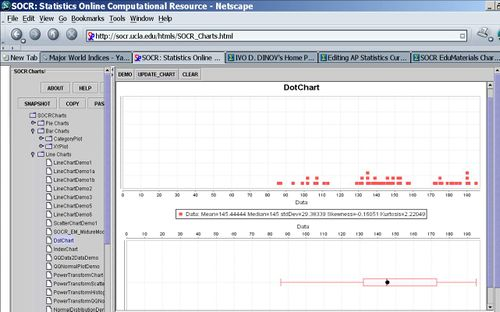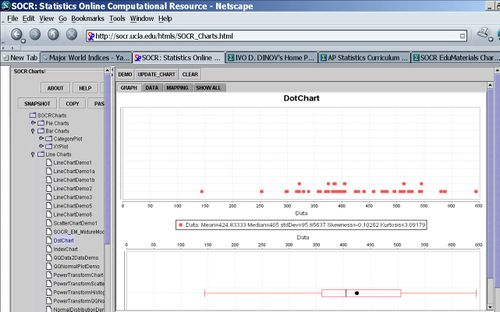# AP Statistics Curriculum 2007 EDA Statistics

## General Advance-Placement (AP) Statistics Curriculum - Statistics

### Definitions

Variables can be summarized using statistics.

• A statistic is a numerical measure (or a function) that describes a characteristic of the sample.
• A parameter is a numerical measure that describes a characteristic of the population. We use statistics to estimate parameters.
• We use sample-statistics to estimate/understand population parameters or characteristics!
• Notation:
• Population parameters are typically denoted by lower-case Greek letters (e.g., $$\mu, \sigma, \pi$$, etc.)
• Random variables are always denoted by capital letters (e.g., X, Y, U, W, etc.) and specific measurements (an instance of a data sample) are denoted by lower-case letters (e.g., x, y, u, w, etc.).
• Data (sample) driven estimates (for specific population parameters) are always denoted by a corresponding symbol with an over-line or a hat (e.g., $$\hat{\mu}, \overline{y}, \hat{\sigma}, \hat{p}$$, etc.)

### Example

Suppose we are interested in the mean calorie or mean sodium content of hot dogs. It would be difficult to obtain measures of calorie and salt content for ALL hot-dogs. However, we can use the Hot dogs data file that we discussed earlier. This data contains a sample of 54 major hot dog brands. Some of the interesting population parameters are the calorie-mean, sodium-mean, calorie-variance and sodium-variance between hot-dogs. These measures may be estimated from the sample using the corresponding sample-statistics (sample-averages and sample-variances).

• The dot-plot of the Calorie content of all hotdogs is shown in the image below. Notice the summary statistics of mean and standard deviation below the graph!• The dot-plot of the Sodium content of all hotdogs is shown in the image below. Notice the summary statistics of mean and standard deviation below the graph!